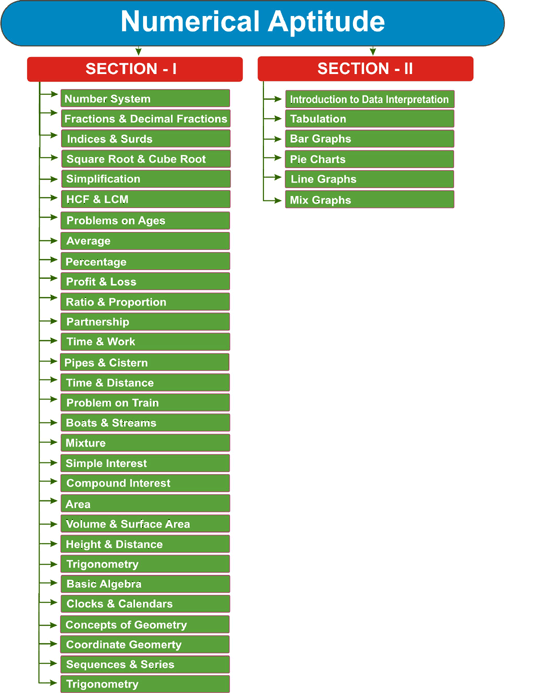NEW!# (Guidance Programme) Combined Graduate Level Examination - Tier - I : Numerical Aptitude

## Numerical Aptitude

The questions will be designed to test the ability of appropriate use of numbers and number sense of the candidate.

The scope of the test will be computation of whole numbers, decimals, fractions and relationships between numbers, Percentage. Ratio & Proportion, Square roots, Averages, Interest, Profit and Loss, Discount, Partnership Business, Mixture and Alligation, Time and distance, Time & Work, Basic algebraic identities of School Algebra & Elementary surds, Graphs of Linear Equations, Triangle and its various kinds of centres, Congruence and similarity of triangles, Circle and its chords, tangents, angles subtended by chords of a circle, common tangents to two or more circles, Triangle, Quadrilaterals, Regular Polygons , Circle, Right Prism, Right Circular Cone, Right Circular Cylinder, Sphere, Hemispheres, Rectangular Parallelepiped, Regular Right Pyramid with triangular or square base, Trigonometric ratio, Degree and Radian Measures, Standard Identities, Complementary angles, Heights and Distances, Histogram, Frequency polygon, Bar diagram & Pie chart.